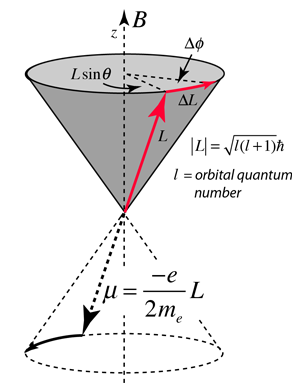# Larmor PrecessionWhen a magnetic moment μ is placed in a magnetic field B, it experiences a torque which can be expressed in the form of a vector productFor a static magnetic moment or a classical current loop, this torque tends to line up the magnetic moment with the magnetic field B, so this represents its lowest energy configuration. But if the magnetic moment arises from the motion of an electron in orbit around a nucleus, the magnetic moment is proportional to the angular momentum of the electron. The torque exerted then produces a change in angular momentum which is perpendicular to that angular momentum, causing the magnetic moment to precess around the direction of the magnetic field rather than settle down in the direction of the magnetic field. This is called Larmor precession.

When a torque is exerted perpendicular to the angular momentum L, it produces a change in angular momentum ΔL which is perpendicular to L, causing it to precess about the z axis. Labeling the precession angle as φ, we can describe the effect of the torque as follows:The precession angular velocity (Larmor frequency) isThese relationships for a finite current loop extend to the magnetic dipoles of electron orbits and to the intrinsic magnetic moment associated with electron spin. There is also a characteristic Larmor frequency for nuclear spins.

In the case of the electron spin precession, the angular frequency associated with the spin transition is usually written in the general formwhere γ is called the gyromagetic ratio (sometimes the magnetogyric ratio) and g is the electron spin g-factor. This angular frequency is associated with the "spin flip" or spin transition, involving an energy change of 2μB. An example for magnetic field 1 Tesla follows.The characteristic frequencies associated with electron spin are employed in electron spin resonance (ESR) experiments, and those associated with the nuclear spin in nuclear magnetic resonance (NMR) experiments.

Index

Magnetic force applications

Magnetic field concepts

Reference
Rohlf, Sec 8-4

Larmor precession wiki

 HyperPhysics***** Electricity and Magnetism R Nave
Go Back